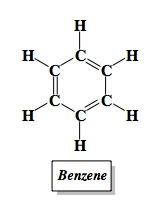## General Chemistry

Learn the toughest concepts covered in Chemistry with step-by-step video tutorials and practice problems by world-class tutors

22. Organic Chemistry

# Hydrocarbon

The term “hydrocarbon” refers to compounds that contain only carbons and hydrogens.

Hydrocarbon Classifications
1
concept

## Identifying Types of Hydrocarbons2m
Play a video:
Hey, guys, In this new video, we're gonna take a look at the concept of hydrocarbons. So hydrocarbons, a term of hydrocarbon refers to compounds that contain Onley, carbons and hydrogen. Remember, hydro in organic chem does not mean water. Hydro actually refers to hydrogen. We're gonna say these compounds may possess single, double or triple bonds. We're gonna say carbons are tetra valid? Remember, I've dropped this word numerous times. Carbon is tetra valid, meaning that when they aren't neutral, they must make four bonds. So when carbon is neutral, it has to make four bonds anyway that it can. There are rare exceptions, or you're going to see a neutral carbon not making four bonds again. Those rare exceptions, you'll see them more so in organic chemistry. When you first take organic one, you'll hear about structures called Khar beans. Car beans are an exception to this, but don't worry. You typically don't learn about car beans until about two thirds of the way into organic one. Now here, al Canes aims. They have nothing but single bonds between their carbon and hydrogen and all. Keen has at least one carbon double bonded toe, another carbon and all kind has at least one carbon triple bonded toe, another carbon and then benzene is this special ring here that we have. So all of these represent different types of hydrocarbons. They only have carbons and hydrogen in them. Now here, knowing that is key to understanding how best to, um, name them what kind of reactions they undergo. Ah, lot of different things. It all starts with being able to identify the different types of hydrocarbons that we have. So, guys, once we've known these four different types, we can then move on toe. All came prefixes, so make sure you come back and take a look at the next video. Where I talk about Al came prefixes.

Hydrocarbons not found in rings are commonly called aliphatic hydrocarbons, while those in rings are commonly referred to as cyclic hydrocarbons.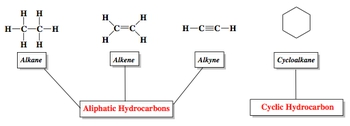Alkane Prefixes
2
concept

## Learning the different alkane prefixes2m
Play a video:
Alright, guys, let's take a look at the Al came prefixes now. So here all came prefixes. So the name of Al Keynes's based on the number of carbons in the compound, these al cane prefixes that go in the front must be memorized in order to name Mawr complex structures later on in the chapter. So we're gonna be dealing with Al Kane's first, then move on to Al Keens and then all kinds, and they're gonna be incorporating things called functional groups, other things that look very different from them. And they're all based initially on memorizing these prefixed names. So we're gonna say here, if you have one carbon in you, you're prefixed. Name is meth, So M E T H math. If you have six, then it's hex. If you have two carbons, then it's F seven. Carbons is helped. Three carbons is pro pope. Eight is Oct. Four is but nine is non 55 is pent and then 10 is deck. So if you have a three carbon chain made up of Onley single bonds, you have an Al cane, A and E. So the name of the Al Kane will be propane. We use the prefixed name and then al Keynes end with a any. That's why it's called propane. So we're gonna see how exactly do we use these prefixes to name Al Canes and then Mawr complicated types of organic molecules. So we've looked at this. We're gonna move on to the next section, so make sure you come back and take a look at the next video and see how else this builds on top of what we learned so far.

The name of alkanes is based on the number of carbons in the compound and each has a set alkane prefix.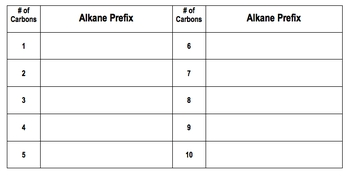Alkanes
3
concept

## Understanding Alkanes2m
Play a video:
Alright, guys. So let's take a look about kings. So here we're gonna say al canes are hydrocarbons that contain Onley single bonds. So there's only single bonds between the carbon and hydrogen. They're sometimes referred simply as saturated hydrocarbons. So saturated means that every single carbon has as many hydrogen as possible on it. So since all the carbons are connected toe four groups, they all have an SP three hybridization. So remember, if you're sp three hybridize, you're connected to four different things whether they be elements or lone pairs. If you don't quite remember, hybridization makes you go back and take a look at my other chapter videos. Or we talk about hybridization and how it relates to lewis dot structures. Now this is important. Al Keynes have a generic formula. Their genetic formula is C N h two n plus two where n is the number of carbons. So here remember, we said on the last page that the Al came prefix when you have two carbons is F and because this is the only single bonds between the carbon and hydrogen, it's an al cane am E. That's why the ending is aimed. And if we use this formula here. Ethane has two carbons in it, so and it's too. So if you work it out, it's C two h six, and that's a things formula here. If you have a three, if you have three carbons. We said that your prefix name was pro pop and it's single bonds. It's an al cane. That's why the ending is a name. And then if we use that formula B C. Three h two times three plus two So pro pains formula is C three h eight so we can use this formula determined. Do we have an Al cane or not? Do we have an AL Keen or Al kind? Now that we've seen the basics in terms of this, we could take a look at what's going on in terms of figuring out the formula of a compound that's made up of only single bonds and eight carbons. So I want you guys to attempt to name this example here, come back and see how I answer it and compare your answer to my answer

Alkanes are hydrocarbons that contain only single bonds. They are sometimes referred to simply as “ saturated” hydrocarbons.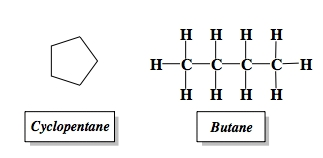4
example

## Determine the formula and name of a hydrocarbon that contains only single bonds and 8 carbons2m
Play a video:
Alright, guys, let's take a look at that example that was left for us to do so. Here, it says determine the formula and name of Ah, hydrocarbon that contains Onley, single bonds and eight carbons. So we know it has only single bonds. So it's an Al cane, which means the end of the name of the pain. Eight carbons. We're gonna say the formula B C N h two n plus two. So we're gonna say here that this would be C eight h two times eight plus two So that b c h h 18. So that would be the molecular formula C eight h 18. And then we would say here that if you have a carbons, your prefixes oct And since it's now came the ending his aim So it be octane. This would be the general generic name of this eight carbon al cane chain. Now here we can say there is a direct relationship between Al Canes. Wait and it's measured. Um, it's measured boiling point. We're gonna say generally the greater the molecular weight of it all cane, the higher its boiling point will be. Remember this deals with inter molecular forces we talk about London dispersion forces. These are all hydrocarbons are all non polar. If you're non poll, your inter molecular force is London dispersion and one of the big factors that increases London dispersion Strength is mass. So if you take a look, you're gonna notice that the lighter, high hydrocarbons, the light around canes up to four. They exist as gasses, meaning that they're incredibly easy to vaporize here. As we keep going down, they're gonna transition from liquids and volatile just means that reacts very easily. Heating it up slightly causes immediate change. So here we're going from liquids toe liquid with very high boiling point. Why? Because the masses increasing as we have more and more carbons until we finally get to a point where we have over 50 carbons and then these falcons finally exists as solids at room temperature. They'll exist mainly as petroleum jelly and waxes. Okay, so petroleum jelly and waxes air just hydrocarbons that have been solidified because they have so many carbons. The weights are large, giving them a stronger inter molecular force London dispersion, which gives it a higher boiling point. So just remember, the greater the mass the greater. The boiling point will be an organic when you get to what you're going to greater detail about other factors that could influence the London dispersion forces before right now, just focus on the Mass.
Alkenes
5
concept

## Understanding Alkenes4m
Play a video:
Alright guys. So let's take a look at the AL keen aspect now of hydrocarbons. So here all kinds are hydrocarbons that contain at least one double bond. Now, once you start adding double bonds which are pi bonds, you become unsaturated unsaturated just refers to the carbon. Not having the maximum amount of hydrogen is possible here. The double bonded carbons are connected to three electron groups. So those double bonded carbons have an SP two hybridization. And now here What we need to realize is if we take a look at F 18 F ethane waas, those two carbons, each one connected to three hydrogen, notice the difference between them in the al cane version. Both carbons have three hydrogen, but once we create that double bond, what happens? We lose two hydrogen as a result. Why? Because here, those carbons can't be allowed to continue. Having three Hydrogen is each. Because then, if we take a look at this carbon, it's making 12345 bonds. Carbon cannot make more than four bonds, so the trade off is this. If you lay down an extra bond, you have to give up a hydrogen and Since we're laying down an extra bomb between these two carbons, they each have to give up one hydrogen. So here, same thing here. It should have had an H year. And actually, it shouldn't have this h here at all. So make sure you do a correction of this. There shouldn't be an h there at all, because that carbon is making five bonds. So we removed that age there, so I'll make sure to correct that on the pdf guys. But make sure that you realize right now that that carbon should not have to bonds on two hydrogen on it should only have one. So let's think about this. We're gonna say here that the formula for an al que nous c n h two n plus two. When we put down a double bomb, we lose two hydrogen. So all the form of an AL Keen be what we lose two ages so that two wouldn't be there anymore. So the generic formula for now keen with only one double bond will be C N h two n. So again, this is the generic formula for an AL Keen with Onley one double bond. But it also represents the formula for cyclo. All came because if we think about it, this cycle walkin, if we look has six carbons in it. Remember every and every corners of carbon, each one we see making to bonds so each carbon has to hydrogen is we don't see, so the formula of this thing would be C six h 12. It has the same exact formula as a now keen ah compound with only one double bond. Why? Because the al cane form should have been C six h two times six plus two, So that have been C six h 14. What's the difference? We lost two hydrogen in order to make a ring. So when you make a double bond, you have to give up to hydrogen. And when you make a ring, you have to give up to hydrogen. That's why they have similar formulas here. This is just the basics in terms of identifying, uh, the formulas for an Al Keenan, a cyclo all cane when you move on to organic and I'm saying that a lot you can get more and more complex types of molecules. We'll see a few today, but just realize that get more and more complex. Now that we've seen this, let's see if you guys can try to answer this question here. So I just need the formula, the simplest possible name of Ah, hydrocarbon that contains one double bond and six carbons. Attempt this on your own and then come back and see how I best approach it.

Alkenes are hydrocarbons that contain at least one double bond. They are sometimes referred to simply as “unsaturated” hydrocarbons.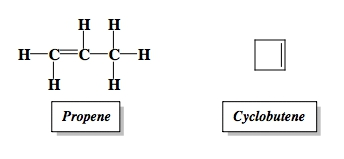6
example

## Determine the formula and simplest name of a hydrocarbon that contains one double bond and 6 carbons1m
Play a video:
Alright guys. So let's take a look at that example that left at the bottom of the page. So here I say determine the formula and simple and simplest name of ah, hydrocarbon that contains one double bond and six carbons. So remember one double bond medicinal keen e in, which means the ending starts with E. Remember, the formula is C N H two n so the formula B C six h two times six. So I d. C six h 12. If you have six carbons, remember, your prefix is hex. And because it has a double bond, it's an AL Keen. So it ends with Dean, So the most simplest way we could name it is Hexen. Now we'll get more details in terms of naming Al Keane's. But for right now, we're just gonna say it's a heck seen later, I will learn that it depends where the double bond is to get the complete name of the Al Keen. But for right now, let's not worry about that. So we've seen Al Keynes. We've seen Al Keane's move on to the next section and take a look at all kinds. It's a common thing that we're going to be seen. So come back, click on the next video and see how I approach the AL kind section.
Alkynes
7
concept

## Understanding Alkynes1m
Play a video:
Hey, guys, let's take a look at the AL kind section now of hydrocarbons. So we say all kinds are hydrocarbons that contain at least one triple bond. They're sometimes referred to simply as unsaturated hydrocarbons, just like the Al Keene's. Now, the triple bonded carbons are connected to two electron groups, so either lone pairs or surrounding elements. Therefore, their hybridization are SP hybridized. Now here we're not laying down one pie bond were laying down two PI bonds to make a triple bond. And remember, every time we put down an extra bond we have to give up to hydrogen is in the process. So if we're putting down to bonds now, two extra bonds, that means that we're losing a total of four hydrogen. Because here, ethane originally had three hydrogen on each carbon. Now we have a triple bond there, so each carbon only gets one each, so we keep minus ing to every tell me at an extra bond. So for an al kind with one triple bond, the generic formula would be H two n minus two. Now notice two carbons, two carbons is F again for the prefix. And because we have a triple bond. The ending is ein, so this would be f fine here. Three carbons, the prefix we pro pope here because it ends with Ein. That's because we have a triple bond. So it's pro pine. The name sound a little bit funny, I know, but this is how we would name them now, now that we've seen this, let's see if you guys can attempt to do this question here. So now we're looking for basically a hydrocarbon. Its generic name. Nothing specific. It has one triple bond and nine carpets. So apply what we've learned so far and try to answer this question here. Come back, click on the next video and see how I best answer that question.

Alkynes are hydrocarbons that contain at least one triple bond. They are sometimes referred to simply as “unsaturated” hydrocarbons just like the alkenes.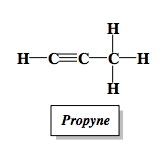8
example

## Determine the formula and simplest name of a hydrocarbon that contains one triple bond and 9 carbons2m
Play a video:
Alright, guys, let's attempt to answer the question that was left at the bottom of the page. So here we say, determine the formula and simplest name of, Ah, hydrocarbon that contains one triple bond and nine carbons. So it has a triple bond, which is it means it's an Al Kaine, which means that the ending will be yien y any now here, and I'll kind with one. Triple bond has the formula of CNN H two n minus two, so c nine h, two times nine minus two means we have C nine h 16. So that's our formula. And then remember, we say If you have nine carbons, your prefix is non so the name will be No. Nine. No nine, okay or known in basically depends on higher. Professor pronounces it so different Professors pronounce it differently. So remember the differences between an al cane and all keen and an al kind. Remember, adding a pie bond removes two hydrogen, which affects the original formula of the Al Cane, which was C. N. H. Two n plus two. Now, now that we've seen these first three types of hydrocarbons, that leaves us with last type now before we go there. What we need to realize here is that if we have an Al Cane and all kind and and all keen that are not in a ring, so you're gonna say non cyclic hydrocarbons are referred to as Al Emphatic hydrocarbons. Okay, so that's a l i p h a t. I see Al Emphatic hydrocarbons. They're not in a ring. So once we start putting these in rings, they may become cyclic hydrocarbons. And the last major one that we're gonna look at are the aromatic hydrocarbons. We're gonna see what key feature do they have in common and what's so special about them. So move onto the next video, guys. Take a look and see what I talk about in terms off aromatic hydrocarbons.
Aromatic Hydrocarbons
9
concept

## Understanding Aromatic Hydrocarbons5m
Play a video: